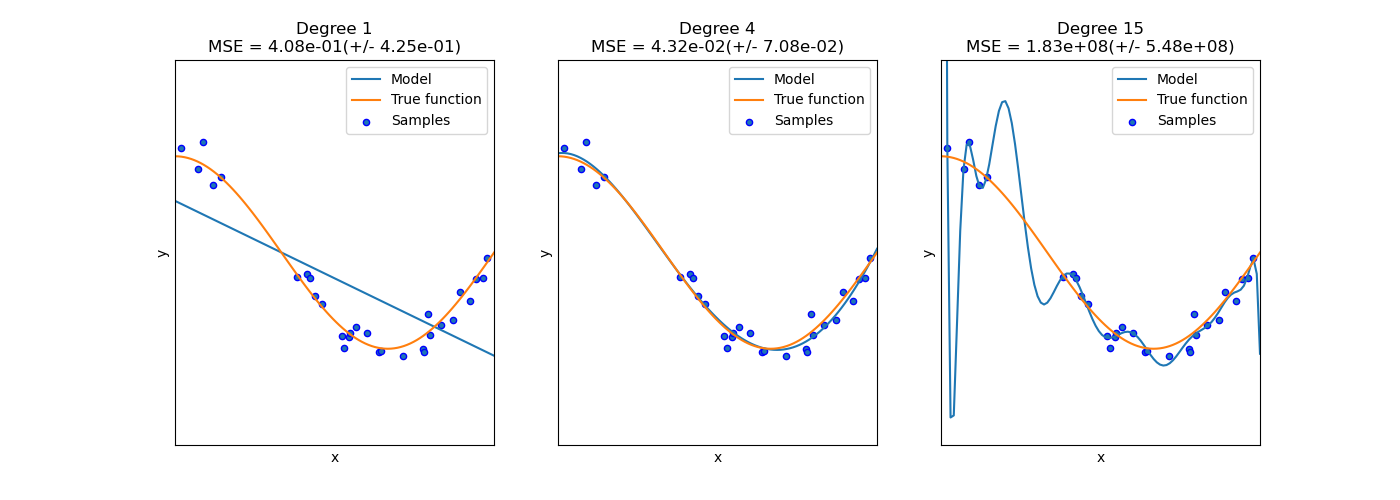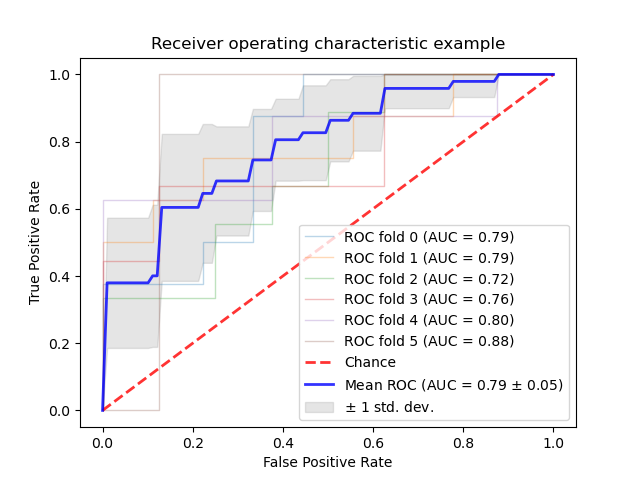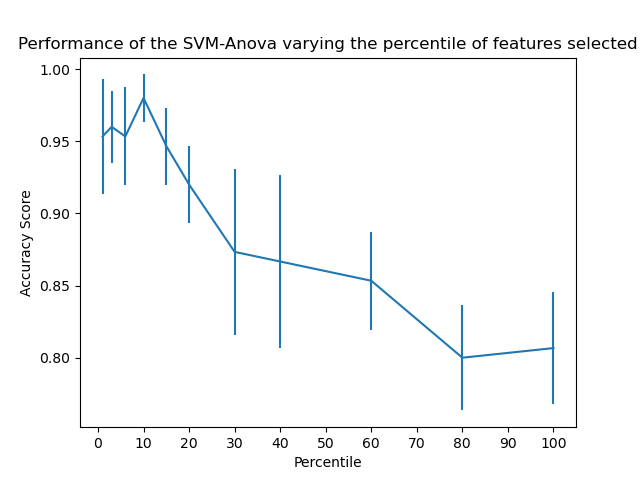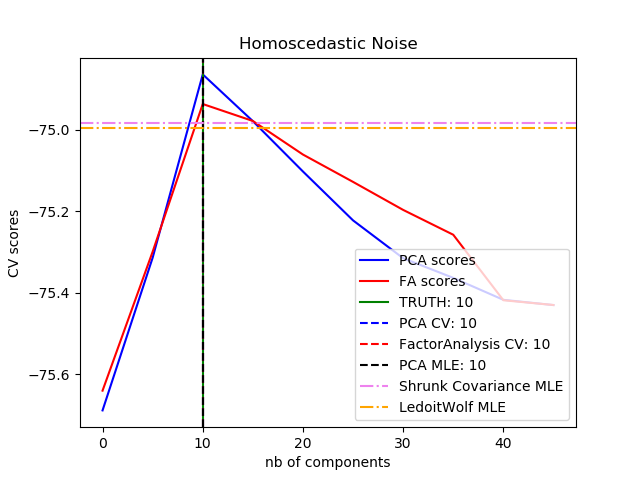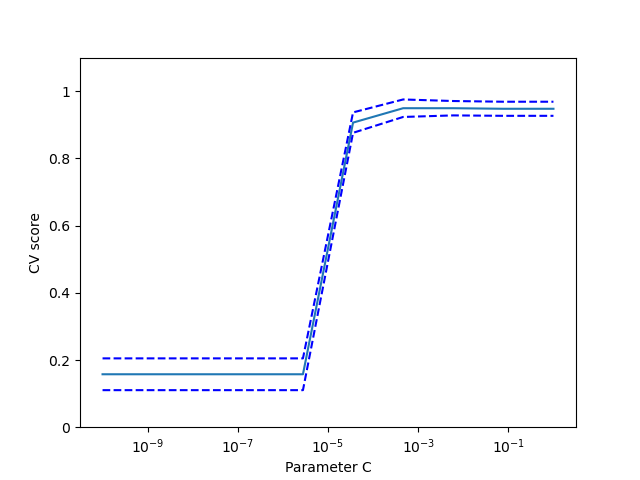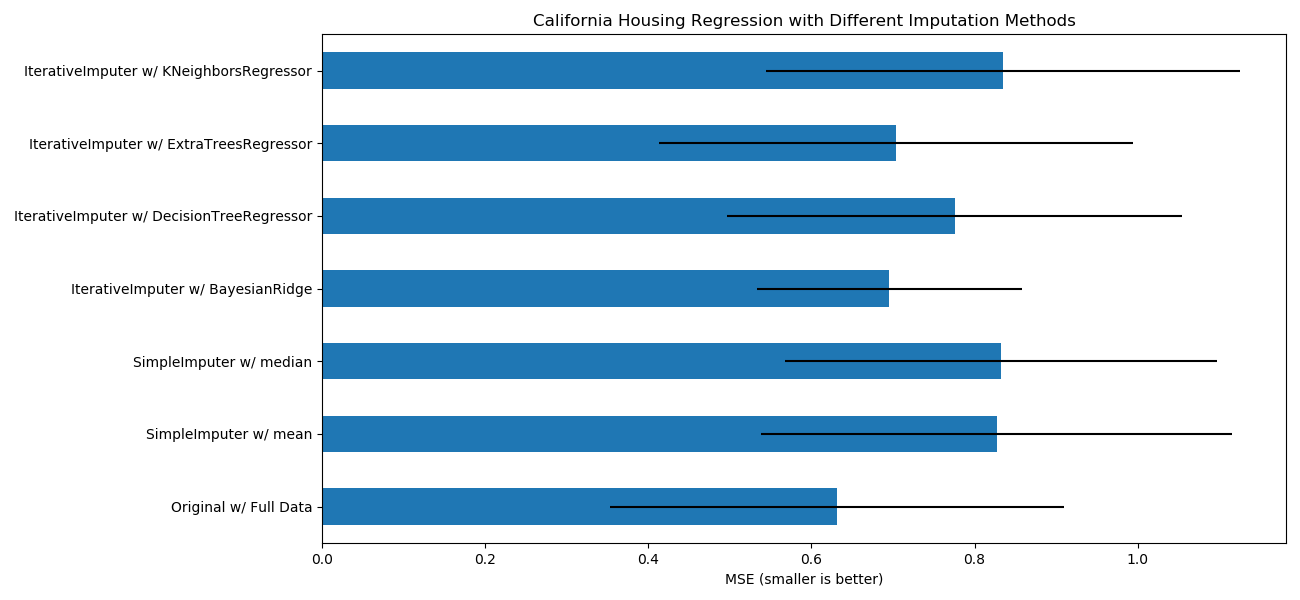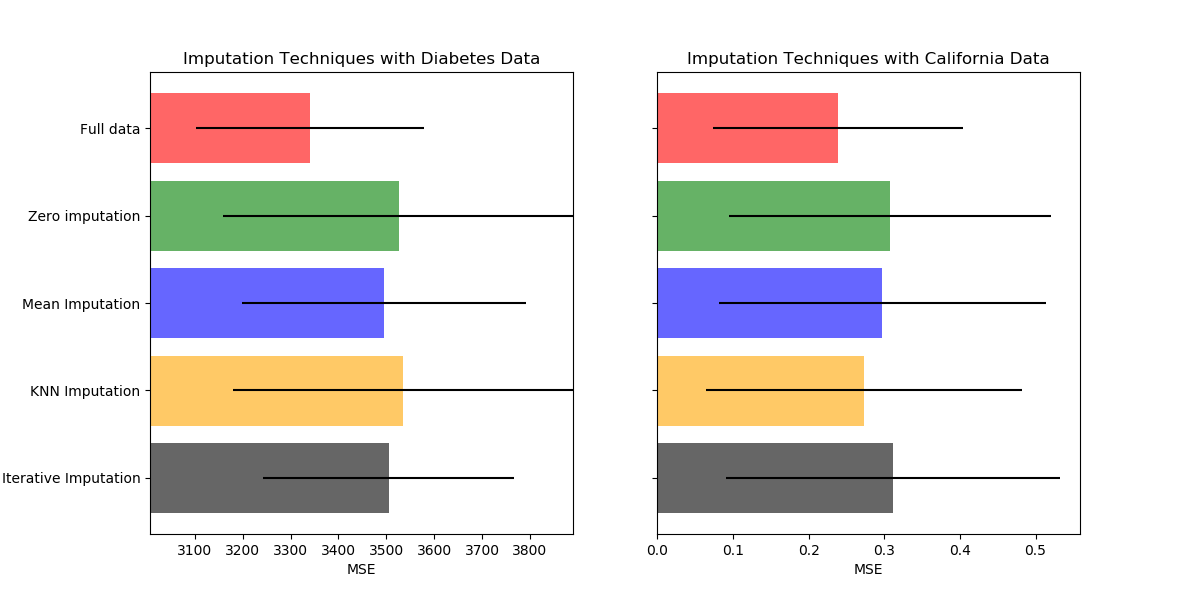# sklearn.model_selection.cross_val_score¶

sklearn.model_selection.cross_val_score(estimator, X, y=None, *, groups=None, scoring=None, cv=None, n_jobs=None, verbose=0, fit_params=None, pre_dispatch='2*n_jobs', error_score=nan)

[源码]

estimator estimator object implementing ‘fit’

X array-like of shape (n_samples, n_features)

y array-like of shape (n_samples,) or (n_samples, n_outputs), default=None

groups array-like of shape (n_samples,), default=None

scoring 一个str(参见模型评估文档)或一个信息为scorer(estimator, X, y)的可调用对象或函数的评分器，它应该只返回一个值。

cross_validate类似， 但只允许使用一个指标。

cv int, cross-validation generator or an iterable, default=None

- None，默认使用5折交叉验证
- int，用于指定(Stratified)KFold的折数
- CV splitter,
- 可迭代输出训练集和测试集的切分作为索引数组

n_jobs int, default=None

verbose int, default=0

fit_params dict, default=None

pre_dispatch int or str, default=’2*n_jobs’

- None,在这种情况下，所有CPU内核都将立即创建并产生，使它进行轻量级和快速运行的任务，以避免因按需生成作业而造成延迟
-int,给出所产生的总CPU内核数的确切数量
-str,根据n_jobs给出表达式，如'2*n_jobs'
error_score ‘raise’ or numeric, default=np.nan

0.20版中的新功能。

scores array of float, shape=(len(list(cv)),)

sklearn.model_selection.cross_validate 在多个指标上进行交叉验证，并返回训练集准确率、拟合时间和评分时间。

sklearn.model_selection.cross_val_predict 从交叉验证的每个切分中获取预测，用于诊断。

sklearn.metrics.make_scorer 根据绩效指标或损失函数确定评分器。

>>> from sklearn import datasets, linear_model>>> from sklearn.model_selection import cross_val_score>>> diabetes = datasets.load_diabetes()>>> X = diabetes.data[:150]>>> y = diabetes.target[:150]>>> lasso = linear_model.Lasso()>>> print(cross_val_score(lasso, X, y, cv=3))[0.33150734 0.08022311 0.03531764]

## sklearn.model_selection.cross_val_score使用示例¶